## The Local-to-Global Principle of Hasse and Minkowski

Section 16.2.3 might have convinced you that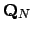is a bizarre pathology. In fact,is omnipresent in number theory, as the following two fundamental examples illustrate.

In the statement of the following theorem, a to a homogeneous polynomial equation is a solution where not all indeterminates are 0.

Theorem 16.2.14 (Hasse-Minkowski)   The quadratic equation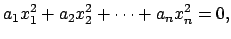(16.1)

with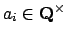, has a nontrivial solution with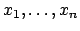in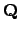if and only if (16.2.1) has a solution in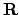and in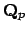for all primes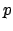.

This theorem is very useful in practice because the-adic condition turns out to be easy to check. For more details, including a complete proof, see [Ser73, IV.3.2].

The analogue of Theorem 16.2.14 for cubic equations is false. For example, Selmer proved that the cubic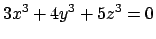has a solution other than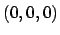inand infor all primesbut has no solution other thanin(for a proof see [Cas91, §18]).

Open Problem. Give an algorithm that decides whether or not a cubic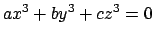has a nontrivial solution in.

This open problem is closely related to the Birch and Swinnerton-Dyer Conjecture for elliptic curves. The truth of the conjecture would follow if we knew that Shafarevich-Tate Groups'' of elliptic curves were finite.

William Stein 2004-05-06